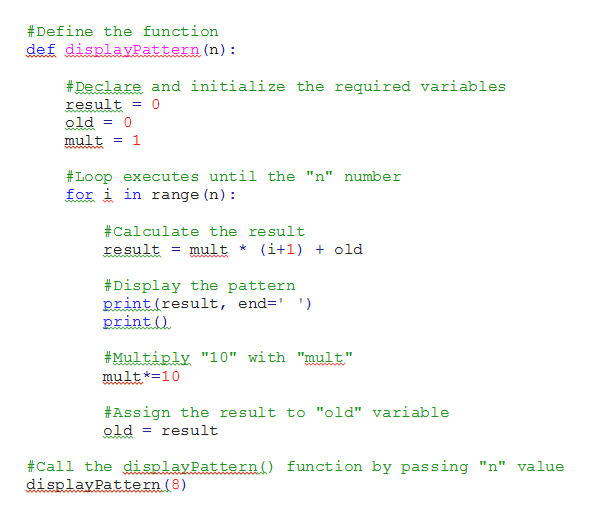# Question: Write a function to display a pattern as follows:12       1......n       n-1             2       1 Book: Introduction to programming using PythonAuthor: Y. Daniel LiangQuestion: Chapter6, Page204, Question: 6.6

Question
101 views

Question: Write a function to display a pattern as follows:

1

2       1

...

...

n       n-1             2       1

Book: Introduction to programming using Python

Author: Y. Daniel Liang

Question: Chapter6, Page204, Question: 6.6

check_circle

Step 1

Solution:

The python program has the following algorithm:

In the displayPattern() function,

• Declare and initialize the required variables.
• The for loop executes until the “n” number. If yes,
• Calculate the result
• Display the pattern.

Call the displayPattern() function by passing "n" value.

Step 2

Screenshot of program:help_outlineImage Transcriptionclose#Define the function def displavPattern (n) #Declare and initialize the required variables result = 0 0 mult 1 #Loop executes until the "n" number for iin range (n) #Calculate the result (i+1) old result mult #Display the pattern print (result, end=' ') print #Multiply "10" with "mult" mult*10 #Assign the result to "old" variable old result = #Call the displayPattern () function by passing "n" value displayPattern (8) fullscreen
Step 3

Editable code:

#Define the function

def displayPattern(n):

#Declare and initialize the required variables

result = 0

old = 0

mult = 1

#Loop executes until the "n" number

for i in range(n):

#Calculate the result

result = mult * (i+1) + old

#Display the pattern

...

### Want to see the full answer?

See Solution

#### Want to see this answer and more?

Solutions are written by subject experts who are available 24/7. Questions are typically answered within 1 hour.*

See Solution
*Response times may vary by subject and question.
Tagged in

### Python